## Radar Waveform Classification Using Deep Learning

This example shows how to classify radar waveform types of generated synthetic data using the Wigner-Ville distribution (WVD) and a deep convolutional neural network (CNN).

Modulation classification is an important function for an intelligent receiver. Modulation classification has numerous applications, such as cognitive radar and software-defined radio. Typically, to identify these waveforms and classify them by modulation type it is necessary to define meaningful features and input them into a classifier. While effective, this procedure can require extensive effort and domain knowledge to yield an accurate classification. This example explores a framework to automatically extract time-frequency features from signals and perform signal classification using a deep learning network.

The first part of this example simulates a radar classification system that synthesizes three pulsed radar waveforms and classifies them. The radar waveforms are:

• Rectangular

• Linear frequency modulation (LFM)

• Barker Code

A radar classification system does not exist in isolation. Rather, it resides in an increasingly occupied frequency spectrum, competing with other transmitted sources such as communications systems, radio, and navigation systems. The second part of this example extends the network to include additional communication modulation types. In addition to the first set of radar waveforms, the extended network synthesizes and identifies these communication waveforms:

• Gaussian frequency shift keying (GFSK)

• Continuous phase frequency shift keying (CPFSK)

• Double sideband amplitude modulation (DSB-AM)

• Single sideband amplitude modulation (SSB-AM)

This example primarily focuses on radar waveforms, with the classification being extended to include a small set of amplitude and frequency modulation communications signals. See Modulation Classification with Deep Learning (Communications Toolbox) for a full workflow of modulation classification with a wide array of communication signals.

Generate 3000 signals with a sample rate of `100` MHz for each modulation type. Use `phased.RectangularWaveform` for rectangular pulses, `phased.LinearFMWaveform` for LFM, and `phased.PhaseCodedWaveform` for phase coded pulses with Barker code.

Each signal has unique parameters and is augmented with various impairments to make it more realistic. For each waveform, the pulse width and repetition frequency will be randomly generated. For LFM waveforms, the sweep bandwidth and direction are randomly generated. For Barker waveforms, the chip width and number are generated randomly. All signals are impaired with white Gaussian noise using the `awgn` function with a random signal-to-noise ratio in the range of [–6, 30] dB. A frequency offset with a random carrier frequency in the range of `[Fs/6`, `Fs/5]` is applied to each signal using the `comm.PhaseFrequencyOffset` object. Lastly, each signal is passed through a multipath Rician fading channel, `comm.RicianChannel`.

The provided helper function `helperGenerateRadarWaveforms` creates and augments each modulation type.` `

```rng default [wav, modType] = helperGenerateRadarWaveforms();```

Plot the Fourier transform for a few of the LFM waveforms to show the variances in the generated set.

```idLFM = find(modType == "LFM",3); nfft = 2^nextpow2(length(wav{1})); f = (0:(nfft/2-1))/nfft*100e6; figure subplot(1,3,1) Z = fft(wav{idLFM(1)},nfft); plot(f/1e6,abs(Z(1:nfft/2))) xlabel('Frequency (MHz)');ylabel('Amplitude');axis square subplot(1,3,2) Z = fft(wav{idLFM(2)},nfft); plot(f/1e6,abs(Z(1:nfft/2))) xlabel('Frequency (MHz)');ylabel('Amplitude');axis square subplot(1,3,3) Z = fft(wav{idLFM(3)},nfft); plot(f/1e6,abs(Z(1:nfft/2))) xlabel('Frequency (MHz)');ylabel('Amplitude');axis square```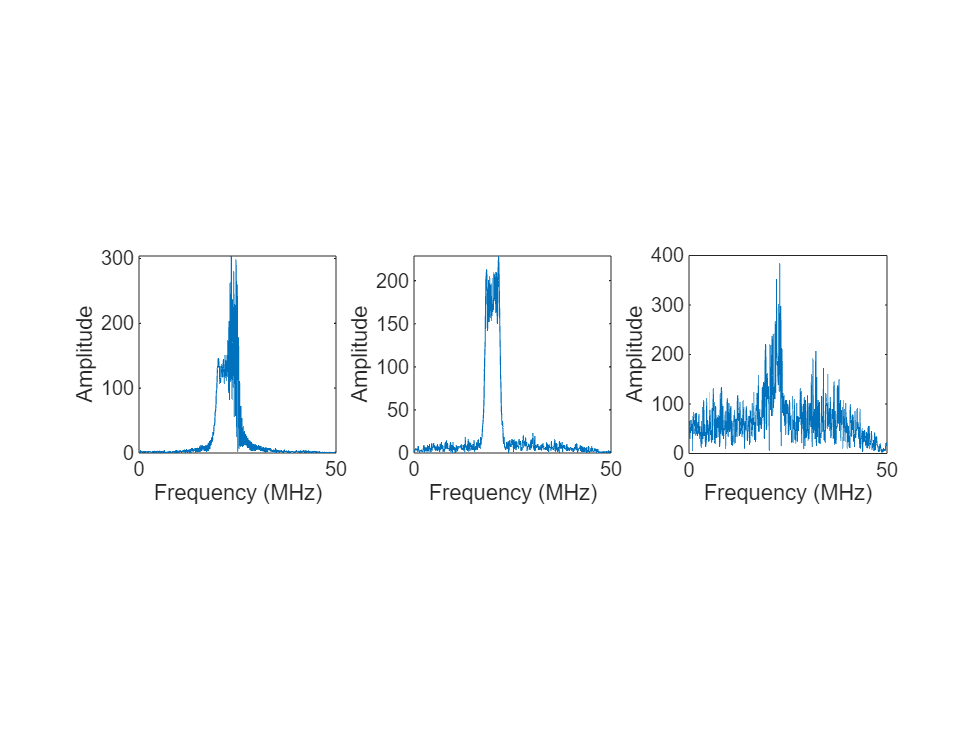### Feature Extraction Using Wigner-Ville Distribution

To improve the classification performance of machine learning algorithms, a common approach is to input extracted features in place of the original signal data. The features provide a representation of the input data that makes it easier for a classification algorithm to discriminate across the classes. The Wigner-Ville distribution represents a time-frequency view of the original data that is useful for time varying signals. The high resolution and locality in both time and frequency provide good features for the identification of similar modulation types. Use the `wvd` function to compute the smoothed pseudo WVD for each of the modulation types.

```figure subplot(1,3,1) wvd(wav{find(modType == "Rect",1)},100e6,'smoothedPseudo') axis square; colorbar off; title('Rect') subplot(1,3,2) wvd(wav{find(modType == "LFM",1)},100e6,'smoothedPseudo') axis square; colorbar off; title('LFM') subplot(1,3,3) wvd(wav{find(modType == "Barker",1)},100e6,'smoothedPseudo') axis square; colorbar off; title('Barker')```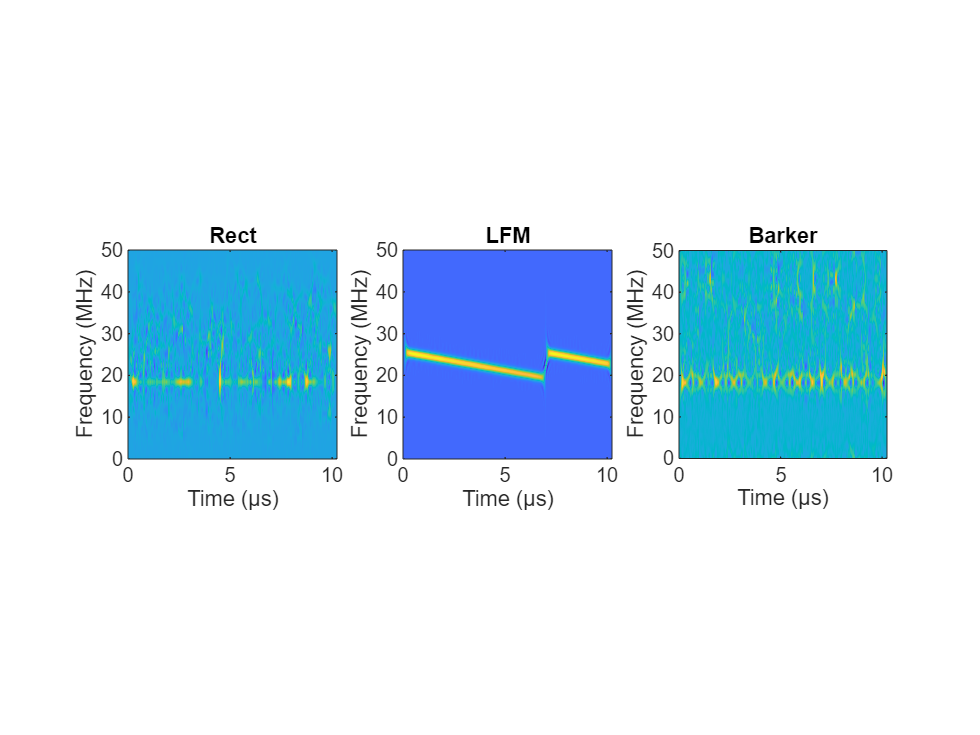To store the smoothed-pseudo Wigner-Ville distribution of the signals, first create the directory `TFDDatabase` inside your temporary directory `tempdir`. Then create subdirectories in `TFDDatabase` for each modulation type. For each signal, compute the smoothed-pseudo Wigner-Ville distribution, and downsample the result to a 227-by-227 matrix. Save the matrix is a `.png` image file in the subdirectory corresponding to the modulation type of the signal. The helper function `helperGenerateTFDfiles` performs all these steps. This process will take several minutes due to the large database size and the complexity of the `wvd `algorithm. You can replace `tempdir` with another directory where you have write permission.

```parentDir = tempdir; dataDir = 'TFDDatabase'; helperGenerateTFDfiles(parentDir,dataDir,wav,modType,100e6)```

Create an image datastore object for the created folder to manage the image files used for training the deep learning network. This step avoids having to load all images into memory. Specify the label source to be folder names. This assigns each signal's modulation type according to the folder name.

```folders = fullfile(parentDir,dataDir,{'Rect','LFM','Barker'}); imds = imageDatastore(folders,... 'FileExtensions','.png','LabelSource','foldernames','ReadFcn',@readTFDForSqueezeNet);```

The network is trained with 80% of the data and tested on with 10%. The remaining 10% is used for validation. Use the `splitEachLabel` function to divide the `imageDatastore` into training, validation, and testing sets.

`[imdsTrain,imdsTest,imdsValidation] = splitEachLabel(imds,0.8,0.1);`

### Set Up Deep Learning Network

Before the deep learning network can be trained, define the network architecture. This example utilizes transfer learning SqueezeNet, a deep CNN created for image classification. Transfer learning is the process of retraining an existing neural network to classify new targets. This network accepts image input of size 227-by-227-by-3. Prior to input to the network, the custom read function `readTFDForSqueezeNet` will transform the two-dimensional time-frequency distribution to an RGB image of the correct size. SqueezeNet performs classification of 1000 categories in its default configuration.

Load SqueezeNet. If Deep Learning Toolbox™ for SqueezeNet Network support package is not installed, the software provides a link to the required support package in the Add-On Explorer. To install the support package, clink the link, and then click Install.

`net = squeezenet;`

Extract the layer graph from the network. Confirm that SqueezeNet is configured for images of size 227-by-227-by-3.

```lgraphSqz = layerGraph(net); lgraphSqz.Layers(1)```
```ans = ImageInputLayer with properties: Name: 'data' InputSize: [227 227 3] Hyperparameters DataAugmentation: 'none' Normalization: 'zerocenter' NormalizationDimension: 'auto' Mean: [1×1×3 single] ```

To tune SqueezeNet for our needs, three of the last six layers need to be modified to classify the three radar modulation types of interest. Inspect the last six network layers.

`lgraphSqz.Layers(end-5:end)`
```ans = 6x1 Layer array with layers: 1 'drop9' Dropout 50% dropout 2 'conv10' Convolution 1000 1x1x512 convolutions with stride [1 1] and padding [0 0 0 0] 3 'relu_conv10' ReLU ReLU 4 'pool10' Average Pooling 14x14 average pooling with stride [1 1] and padding [0 0 0 0] 5 'prob' Softmax softmax 6 'ClassificationLayer_predictions' Classification Output crossentropyex with 'tench' and 999 other classes ```

Replace the 'drop9' layer, the last dropout layer in the network, with a dropout layer of probability 0.6.

```tmpLayer = lgraphSqz.Layers(end-5); newDropoutLayer = dropoutLayer(0.6,'Name','new_dropout'); lgraphSqz = replaceLayer(lgraphSqz,tmpLayer.Name,newDropoutLayer);```

The last learnable layer in SqueezeNet is a 1-by-1 convolutional layer, 'conv10'. Replace the layer with a new convolutional layer with the number of filters equal to the number of modulation types. Also increase the learning rate factors of the new layer.

```numClasses = 3; tmpLayer = lgraphSqz.Layers(end-4); newLearnableLayer = convolution2dLayer(1,numClasses, ... 'Name','new_conv', ... 'WeightLearnRateFactor',20, ... 'BiasLearnRateFactor',20); lgraphSqz = replaceLayer(lgraphSqz,tmpLayer.Name,newLearnableLayer);```

Replace the classification layer with a new one without class labels.

```tmpLayer = lgraphSqz.Layers(end); newClassLayer = classificationLayer('Name','new_classoutput'); lgraphSqz = replaceLayer(lgraphSqz,tmpLayer.Name,newClassLayer);```

Inspect the last six layers of the network. Confirm the dropout, convolutional, and output layers have been changed.

`lgraphSqz.Layers(end-5:end)`
```ans = 6x1 Layer array with layers: 1 'new_dropout' Dropout 60% dropout 2 'new_conv' Convolution 3 1x1 convolutions with stride [1 1] and padding [0 0 0 0] 3 'relu_conv10' ReLU ReLU 4 'pool10' Average Pooling 14x14 average pooling with stride [1 1] and padding [0 0 0 0] 5 'prob' Softmax softmax 6 'new_classoutput' Classification Output crossentropyex ```

Choose options for the training process that ensures good network performance. Refer to the `trainingOptions `documentation for a description of each option.

```options = trainingOptions('sgdm', ... 'MiniBatchSize',128, ... 'MaxEpochs',5, ... 'InitialLearnRate',1e-3, ... 'Shuffle','every-epoch', ... 'Verbose',false, ... 'Plots','training-progress',... 'ExecutionEnvironment','auto',... 'ValidationData',imdsValidation);```

### Train the Network

Use the `trainNetwork` command to train the created CNN. Because of the dataset's large size, the process may take several minutes. If your machine has a GPU and Parallel Computing Toolbox™, then MATLAB automatically uses the GPU for training. Otherwise, it uses the CPU. The training accuracy plots in the figure show the progress of the network's learning across all iterations. On the three radar modulation types, the network classifies almost 100% of the training signals correctly.

`trainedNet = trainNetwork(imdsTrain,lgraphSqz,options);`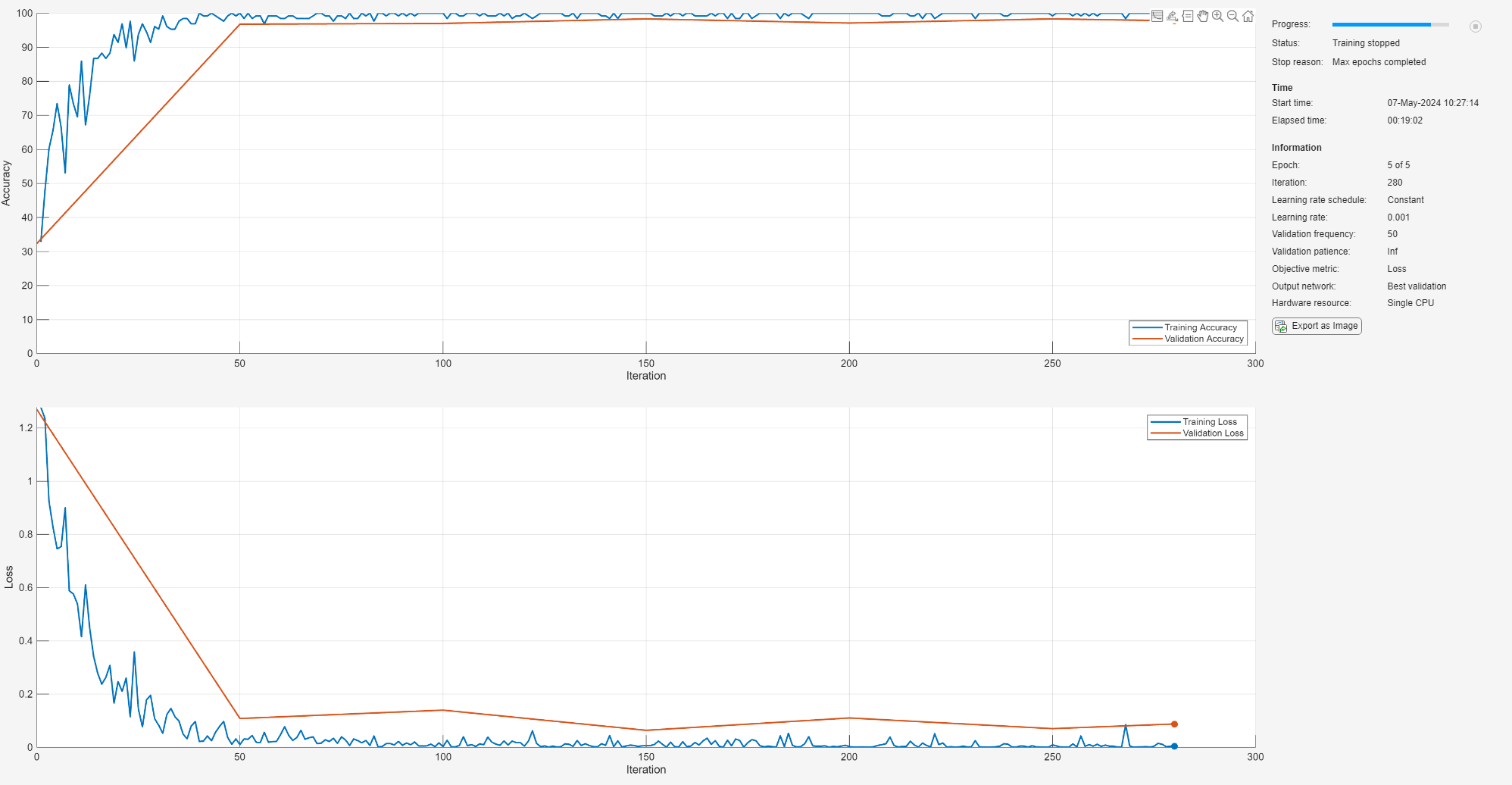### Evaluate Performance on Radar Waveforms

Use the trained network to classify the testing data using the `classify` command. A confusion matrix is one method to visualize classification performance. Use the `confusionchart` command to calculate and visualize the classification accuracy. For the three modulation types input to the network, almost all of the phase coded, LFM, and rectangular waveforms are correctly identified by the network.

```predicted = classify(trainedNet,imdsTest); figure confusionchart(predicted,imdsTest.Labels,'Normalization','column-normalized');```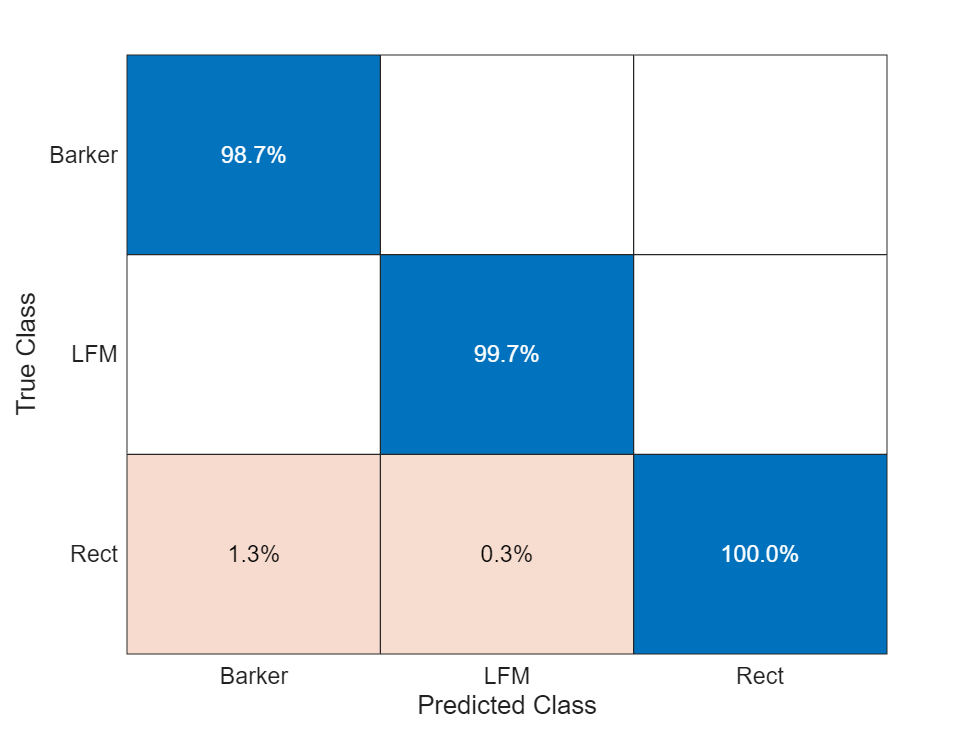### Generate Communications Waveforms and Extract Features

The frequency spectrum of a radar classification system must compete with other transmitted sources. Let's see how the created network extends to incorporate other simulated modulation types. Another MathWorks example, Modulation Classification with Deep Learning (Communications Toolbox), performs modulation classification of several different modulation types using Communications Toolbox™. The helper function `helperGenerateCommsWaveforms` generates and augments a subset of the modulation types used in that example. Since the WVD loses phase information, a subset of only the amplitude and frequency modulation types are used.

See the example link for an in-depth description of the workflow necessary for digital and analog modulation classification and the techniques used to create these waveforms. For each modulation type, use `wvd` to extract time-frequency features and visualize.

```[wav, modType] = helperGenerateCommsWaveforms(); figure subplot(2,3,1) wvd(wav{find(modType == "GFSK",1)},200e3,'smoothedPseudo') axis square; colorbar off; title('GFSK') subplot(2,3,2) wvd(wav{find(modType == "CPFSK",1)},200e3,'smoothedPseudo') axis square; colorbar off; title('CPFSK') subplot(2,3,3) wvd(wav{find(modType == "B-FM",1)},200e3,'smoothedPseudo') axis square; colorbar off; title('B-FM') subplot(2,3,4) wvd(wav{find(modType == "SSB-AM",1)},200e3,'smoothedPseudo') axis square; colorbar off; title('SSB-AM') subplot(2,3,5) wvd(wav{find(modType == "DSB-AM",1)},200e3,'smoothedPseudo') axis square; colorbar off; title('DSB-AM')```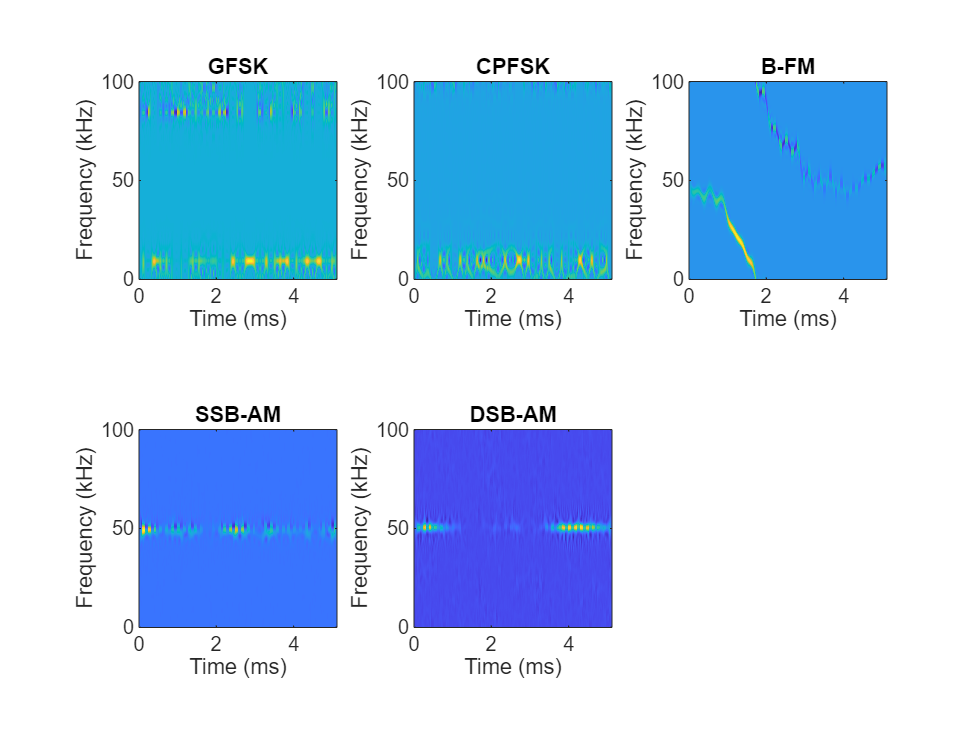Use the helper function `helperGenerateTFDfiles` again to compute the smoothed pseudo WVD for each input signal. Create an image datastore object to manage the image files of all modulation types.

```helperGenerateTFDfiles(parentDir,dataDir,wav,modType,200e3) folders = fullfile(parentDir,dataDir,{'Rect','LFM','Barker','GFSK','CPFSK','B-FM','SSB-AM','DSB-AM'}); imds = imageDatastore(folders,... 'FileExtensions','.png','LabelSource','foldernames','ReadFcn',@readTFDForSqueezeNet);```

Again, divide the data into a training set, a validation set, and a testing set using the `splitEachLabel` function.

```rng default [imdsTrain,imdsTest,imdsValidation] = splitEachLabel(imds,0.8,0.1);```

### Adjust Deep Learning Network Architecture

Previously, the network architecture was set up to classify three modulation types. This must be updated to allow classification of all eight modulation types of both radar and communication signals. This is a similar process as before, with the exception that the `fullyConnectedLayer` now requires an output size of eight.

```numClasses = 8; net = squeezenet; lgraphSqz = layerGraph(net); tmpLayer = lgraphSqz.Layers(end-5); newDropoutLayer = dropoutLayer(0.6,'Name','new_dropout'); lgraphSqz = replaceLayer(lgraphSqz,tmpLayer.Name,newDropoutLayer); tmpLayer = lgraphSqz.Layers(end-4); newLearnableLayer = convolution2dLayer(1,numClasses, ... 'Name','new_conv', ... 'WeightLearnRateFactor',20, ... 'BiasLearnRateFactor',20); lgraphSqz = replaceLayer(lgraphSqz,tmpLayer.Name,newLearnableLayer); tmpLayer = lgraphSqz.Layers(end); newClassLayer = classificationLayer('Name','new_classoutput'); lgraphSqz = replaceLayer(lgraphSqz,tmpLayer.Name,newClassLayer);```

Create a new set of training options.

```options = trainingOptions('sgdm', ... 'MiniBatchSize',150, ... 'MaxEpochs',10, ... 'InitialLearnRate',1e-4, ... 'Shuffle','every-epoch', ... 'Verbose',false, ... 'Plots','training-progress',... 'ExecutionEnvironment','auto',... 'ValidationData',imdsValidation);```

Use the `trainNetwork` command to train the created CNN. For all modulation types, the training converges with an accuracy of about 95% correct classification.

`trainedNet = trainNetwork(imdsTrain,lgraphSqz,options);`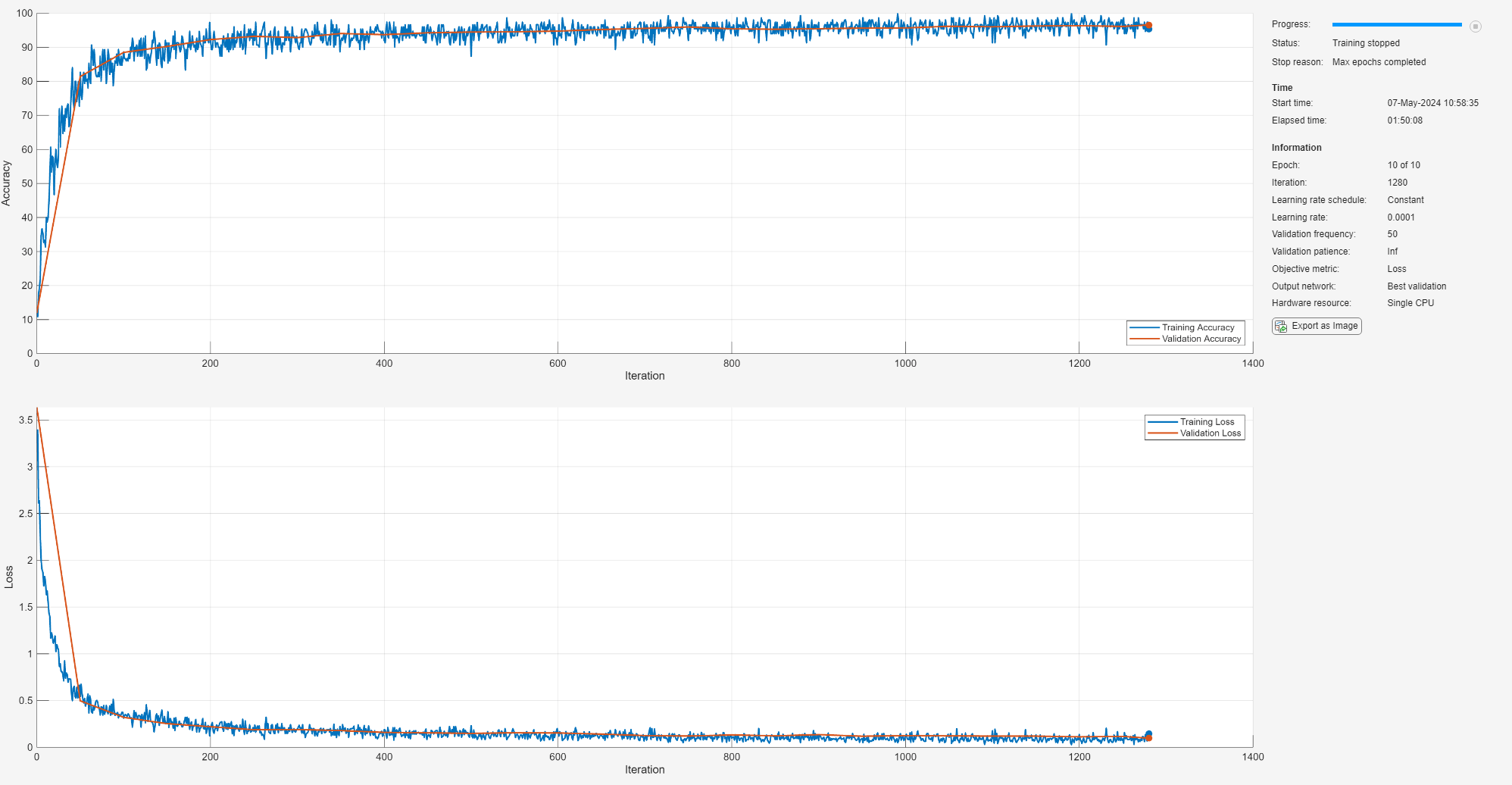### Evaluate Performance on All Signals

Use the `classify` command to classify the signals held aside for testing. Again, visualize the performance using `confusionchart`.

```predicted = classify(trainedNet,imdsTest); figure; confusionchart(predicted,imdsTest.Labels,'Normalization','column-normalized');```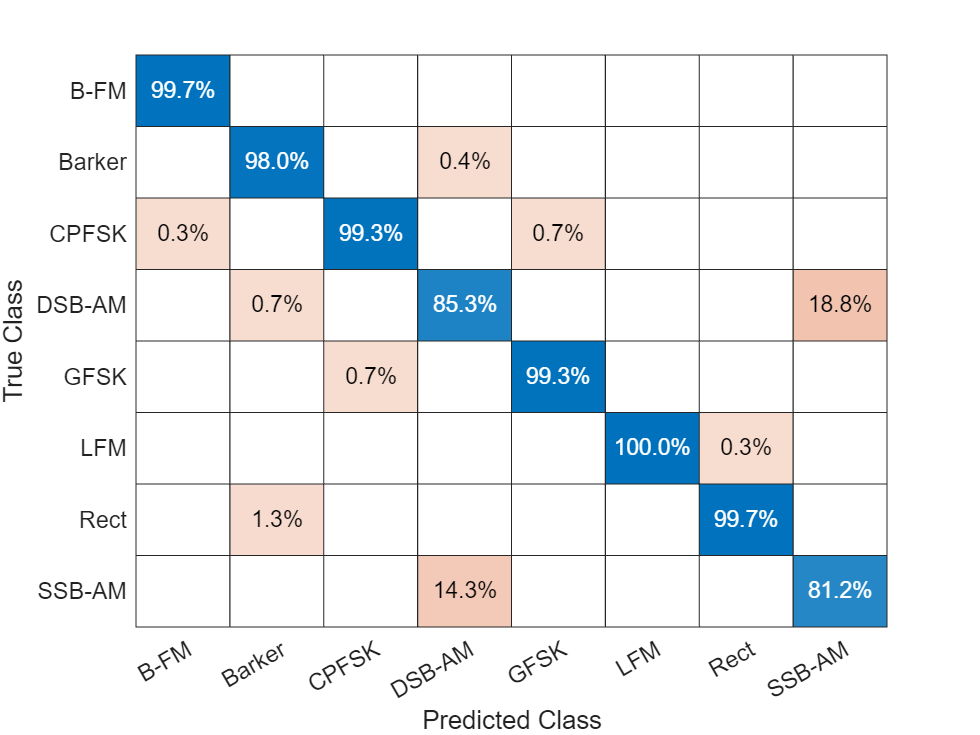For the eight modulation types input to the network, over 99% of B-FM, CPFSK, GFSK, Barker, and LFM modulation types were correctly classified. On average, over 85% of AM signals were correctly identified. From the confusion matrix, a high percentage of SSB-AM signals were misclassified as DSB-AM, and DSB-AM signals as SSB-AM.

Let us investigate a few of these misclassifications to gain insight into the network's learning process. Use the `readimage` function on the image datastore to extract from the test dataset a single image from each class. The displayed WVD visually looks very similar. Since DSB-AM and SSB-AM signals have a very similar signature, this explains in part the network's difficulty in correctly classifying these two types. Further signal processing could make the differences between these two modulation types clearer to the network and result in improved classification.

```DSB_DSB = readimage(imdsTest,find((imdsTest.Labels == 'DSB-AM') & (predicted == 'DSB-AM'),1)); DSB_SSB = readimage(imdsTest,find((imdsTest.Labels == 'DSB-AM') & (predicted == 'SSB-AM'),1)); SSB_DSB = readimage(imdsTest,find((imdsTest.Labels == 'SSB-AM') & (predicted == 'DSB-AM'),1)); SSB_SSB = readimage(imdsTest,find((imdsTest.Labels == 'SSB-AM') & (predicted == 'SSB-AM'),1)); figure subplot(2,2,1) imagesc(DSB_DSB(:,:,1)) axis square; title({'Actual Class: DSB-AM','Predicted Class: DSB-AM'}) subplot(2,2,2) imagesc(DSB_SSB(:,:,1)) axis square; title({'Actual Class: DSB-AM','Predicted Class: SSB-AM'}) subplot(2,2,3) imagesc(SSB_DSB(:,:,1)) axis square; title({'Actual Class: SSB-AM','Predicted Class: DSB-AM'}) subplot(2,2,4) imagesc(SSB_SSB(:,:,1)) axis square; title({'Actual Class: SSB-AM','Predicted Class: SSB-AM'})```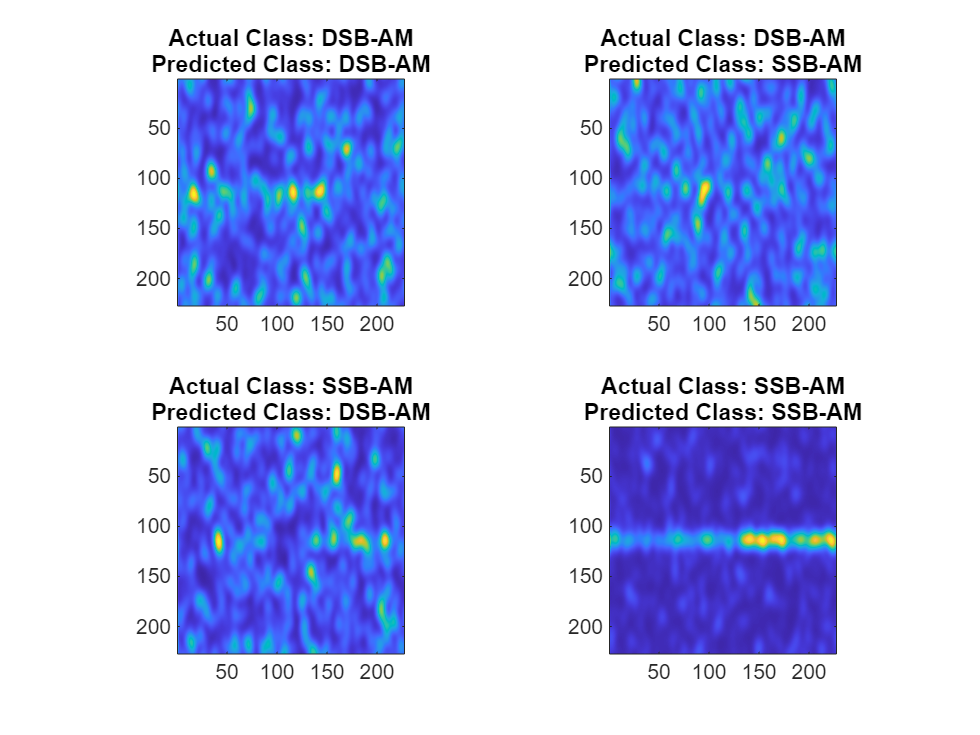### Summary

This example showed how radar and communications modulation types can be classified by using time-frequency techniques and a deep learning network. Further efforts for additional improvement could be investigated by utilizing time-frequency analysis available in Wavelet Toolbox™ and additional Fourier analysis available in Signal Processing Toolbox™.

### References

 Brynolfsson, Johan, and Maria Sandsten. "Classification of one-dimensional non-stationary signals using the Wigner-Ville distribution in convolutional neural networks." 25th European Signal Processing Conference (EUSIPCO). IEEE, 2017.

 Liu, Xiaoyu, Diyu Yang, and Aly El Gamal. "Deep neural network architectures for modulation classification." 51st Asilomar Conference on Signals, Systems and Computers. 2017.

 `Wang, Chao, Jian Wang, and Xudong Zhang. "Automatic radar waveform recognition based on time-frequency analysis and convolutional neural network." IEEE International Conference on Acoustics, Speech and Signal Processing (ICASSP). 2017.